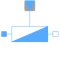# Information

This information is part of the Modelica Standard Library maintained by the Modelica Association.

The admittance model represents a parallel connection of a conductor and either a capacitor or inductor.The linear admittance connects the complex voltage `v` with the complex current `i` by `v*Y = i`. The admittance `Y_ref = G_ref + j*B_ref` is given as complex input signal, representing the resistive and reactive component of the input admittance. The resistive component is modeled temperature dependent, so the real part `G_actual = real(Y)` is determined from the actual operating temperature and the reference input conductance `real(Y_ref)`. The reactive component `B_actual = imag(Y)` is equal to `imag(Y_ref)` if `frequencyDependent = false`. Frequency dependency is considered by `frequencyDependent = true`, distinguishing two cases:

(a) `imag(Y_ref) > 0`: capacitive case
The actual susceptance `B_actual` is proportional to `f/f_ref`
(b) `imag(Y_ref) < 0`: inductive case
The actual susceptance `B_actual` is proportional to `f_ref/f`

#### Note

A zero crossing of the real or imaginary part of the admittance signal `Y_ref` could cause singularities due to the actual structure of the connected network.

# Parameters (6)

useHeatPort Value: false Type: Boolean Description: =true, if heatPort is enabled Value: T_ref Type: Temperature (K) Description: Fixed device temperature if useHeatPort = false Value: 293.15 Type: Temperature (K) Description: Reference temperature Value: 0 Type: LinearTemperatureCoefficient (¹/K) Description: Temperature coefficient of resistance (R_actual = R_ref*(1 + alpha_ref*(heatPort.T - T_ref)) Value: false Type: Boolean Description: Consider frequency dependency, if true Value: 1 Type: Frequency (Hz) Description: Reference frequency, if frequency dependency is considered

# Connectors (4)

pin_p pin_n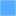Type: PositivePin Description: Positive quasi-static single-phase pin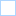Type: NegativePin Description: Negative quasi-static single-phase pin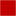Type: HeatPort_a Description: Conditional heat port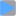Type: ComplexInput Description: Variable complex admittance

# Components (2)

v i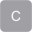Type: ComplexVoltage Description: Complex voltageType: ComplexCurrent Description: Complex current

# Used in Components (1)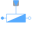VariableAdmittance Modelica.Electrical.QuasiStationary.MultiPhase.Basic Multiphase variable admittance